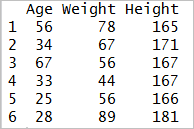# Explain sapply() function?

## sapply() function

sapply() function takes list, vector or data frame as input and gives output in vector or matrix. It is useful for operations on list objects and returns a list object of same length of original set. Sapply function in R does the same job as lapply() function but returns a vector.

sapply(X, FUN) Arguments: -X: A vector or an object -FUN: Function applied to each element of x

We can measure the minimum speed and stopping distances of cars from the cars dataset.

dt ← cars lmn_cars ← lapply(dt, min) smn_cars ← sapply(dt, min) lmn_cars

Output:

smn_cars

Output:

## speed dist ## 4 2

lmxcars ← lapply(dt, max) smxcars ← sapply(dt, max) lmxcars

Output:

smxcars

Output:

## speed dist ## 25 120

We can use a user built-in function into lapply() or sapply(). We create a function named avg to compute the average of the minimum and maximum of the vector.

avg ← function(x) { ( min(x) + max(x) ) / 2} fcars ← sapply(dt, avg) fcars

Output

## speed dist ## 14.5 61.0

Sapply in R is more efficient than lapply() in the output returned because sapply() store values direclty into a vector. In the next example, we will see this is not always the case.

sapply function takes list, vector or Data frame as input. It is similar to lapply function but returns only vector as output.sapply() function does the same jobs as lapply() function but returns a vector.Example for Sapply function in R:

sapply(BMI_df, function(BMI_df) BMI_df/2)

the above Sapply function divides the values in the dataframe by 2 and the output will be in form of vector